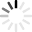# Subtraction Quizzes & Trivia

I suddenly realize I have to answer 5 quizzes. I finished 3. How many am I left with? Hm, not so difficult, is it? Then, how about some of these: what is the Latin name of the square tablet strewn with dust that the Romans used in order to solve difficult subtractions?

Oh wait, let’s not step back from the math in land in the land of history. Here you go then, solve this one 3 – (-2) – 7 – (-8) – 6 =? But still, let’s go back to that history bit for just one more question: what are the two most widely used methods of teaching subtraction to young school age children? Here’s a quick hint: both names of the methods start with “a” (a capital “a”, as in a country name that starts with “a”, get it?!). Now I’m going to leave you to it: happy subtracting!
Top Trending

Check out this math subtraction practice for your kids!

Questions: 10  |  Attempts: 558   |  Last updated: Jan 3, 2013
• Sample Question
20 - 12 =Quiz to show mastery of two-digit subtraction facts for 1st Grade.

Questions: 10  |  Attempts: 587   |  Last updated: Jul 30, 2011
• Sample Question
Another way to explain subtraction isQuestions: 26  |  Attempts: 86   |  Last updated: Feb 16, 2014
• Sample Question
1 + 1 =This is a five minute mixed timed subtraction facts test.

Questions: 99  |  Attempts: 62   |  Last updated: Jan 2, 2013

Are you aware of mathematical calculations? Can you solve the word problems in one go? If yes, then try out this quiz on subtraction. Read the questions carefully and take your time. Practice more if you can't answer...

Questions: 10  |  Attempts: 64   |  Last updated: Feb 12, 2021
• Sample Question
If you have 5 apples and you give 2 away how many do you have left?Related